# NCERT Solutions For Class 11 Maths Chapter 6 Exercise 6.2

Go back to  'Linear Inequalities'

## Chapter 6 Ex.6.2 Question 1

Solve the given inequality graphically in two-dimensional plane: $$x + y < 5$$

### Solution

The graphical representation of $$x + y = 5$$ is given as dotted line in the figure below.

This line divides the xy-plane in two planes I and II

Select a point (not on the line), which lies in one of the half planes, to determine whether the point satisfies the given inequality or not.

We select the point as $$\left( {0,0} \right)$$.

It is observed that,

$$0 + 0 < 5$$ or $$0 < 5,$$which is true

Therefore, half plane II is not the solution region of the given inequality. Also, it is evident that any point on the line does not satisfy the given strict inequality.

Thus, the solution region of the given inequality is the shaded half plane I excluding the points on the line.

This can be represented as follows: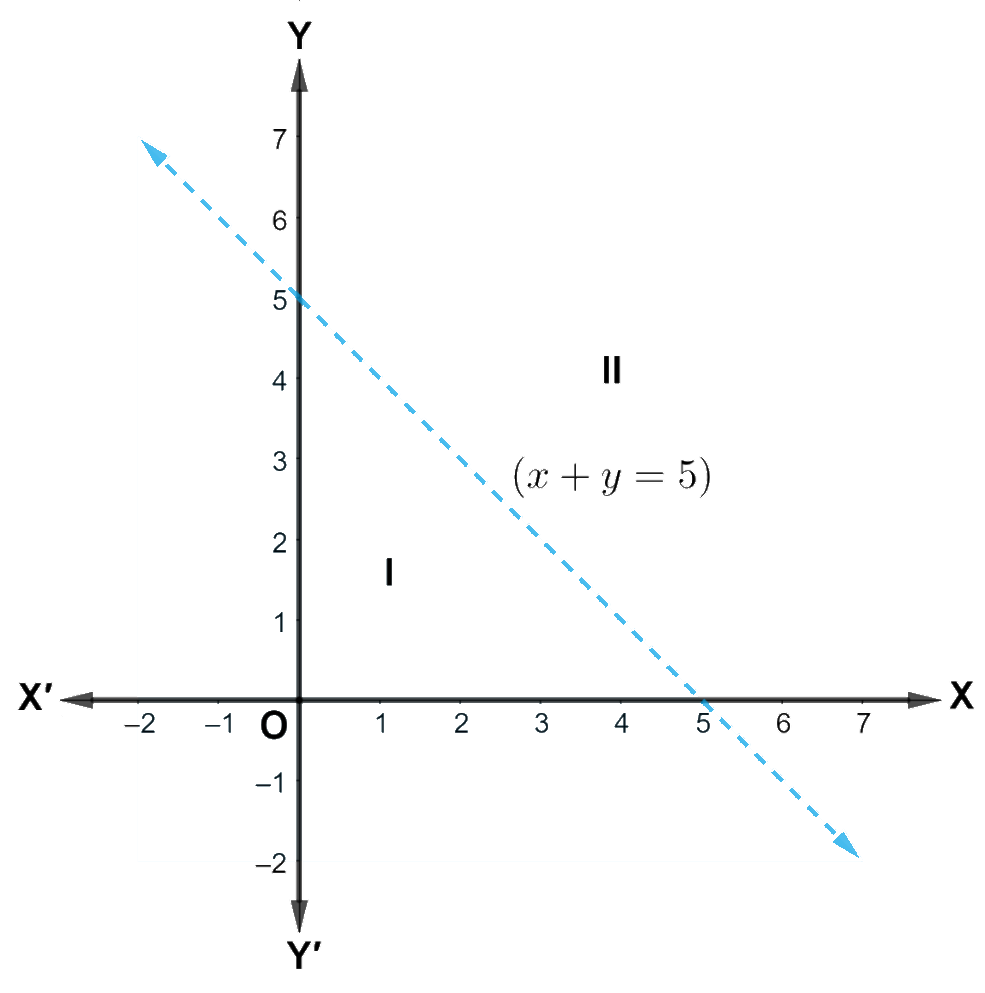## Chapter 6 Ex.6.2 Question 3

Solve the given inequality graphically in two-dimensional plane: $$3x + 4y \le 12$$.

### Solution

The graphical representation of $$3x + 4y = 12$$ is given in the figure below.

This line divides the $$xy-$$plane in two half planes, I and II.

Select a point (not on the line), which lies in one of the half planes, to determine whether the point satisfies the given inequality or not.

We select the point as $$\left( {0,0} \right)$$.

It is observed that,

$$3\left( 0 \right) + 4\left( 0 \right) \le 12$$ or $$0 \le 12,$$which is true

Thus, the solution region of the given inequality is the shaded half plane I, including the points on the line.

This can be represented as follows: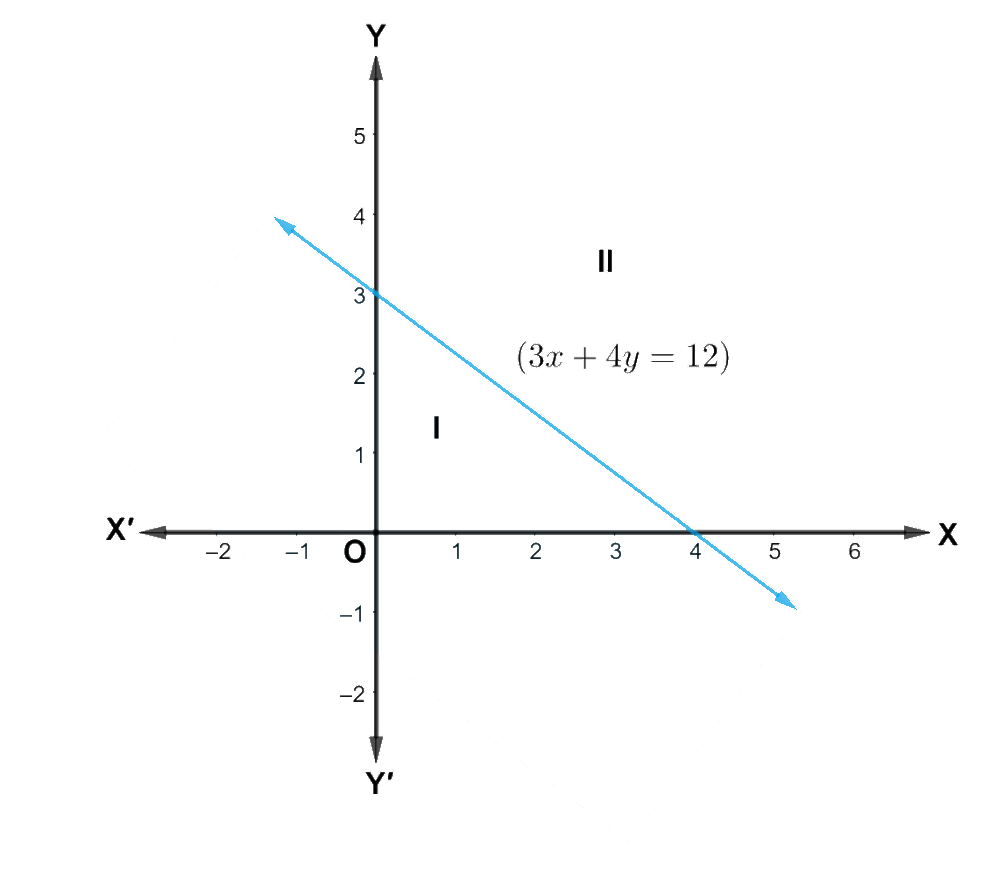## Chapter 6 Ex.6.2 Question 4

Solve the given inequality graphically in two-dimensional plane: $$y + 8 \ge 2x$$

### Solution

The graphical representation of $$y + 8 = 2x$$ is given in the figure below.

This line divides the $$xy-$$plane in two half planes.

Select a point (not on the line), which lies in one of the half planes, to determine whether the point satisfies the given inequality or not.

We select the point as $$\left( {0,0} \right)$$.

It is observed that,

$$0 + 8 \ge 2\left( 0 \right)$$ or $$8 \ge 0,$$ which is true

Therefore, lower half plane is not the solution region of the given inequality. Also, it is evident that any point on the line satisfies the given inequality.

Thus, the solution region of the given inequality is the upper half plane containing the points including the line.

The solution region is represented by the shaded region as follows: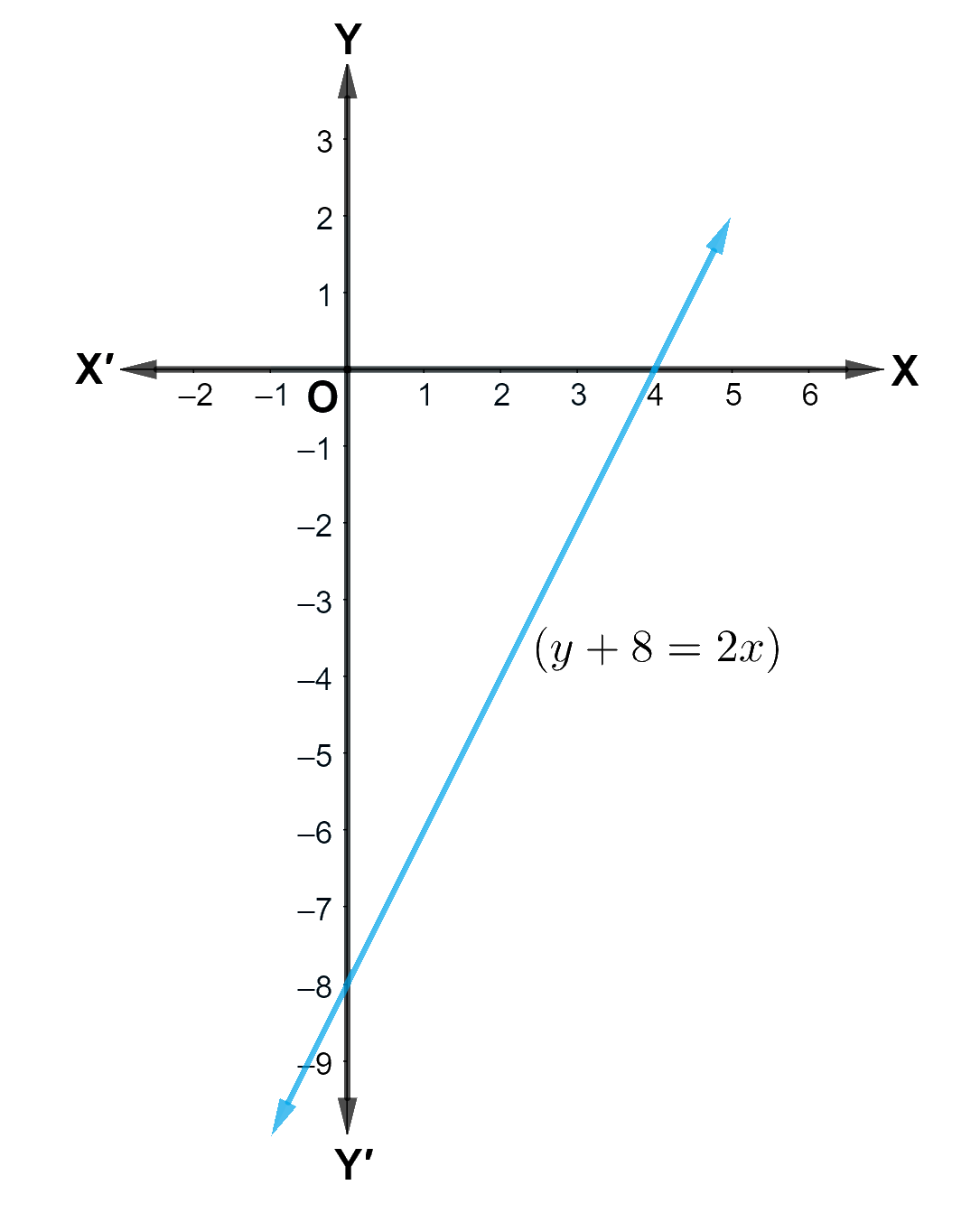## Chapter 6 Ex.6.2 Question 5

Solve the given inequality graphically in two-dimensional plane: $$2x + y \ge 6$$.

### Solution

The graphical representation of $$2x + y = 6$$ is given in the figure below.

This line divides the $$xy-$$plane in two half planes, I and II.

Select a point (not on the line), which lies in one of the half planes, to determine whether the point satisfies the given inequality or not.

We select the point as $$\left( {0,0} \right)$$.

It is observed that,

$$2\left( 0 \right) + 0 \ge 6$$ or $$0 \ge 6,$$which is false

Therefore, half plane I is not the solution region of the given inequality. Also, it is evident that any point on the line satisfies the given inequality.

Thus, the solution region of the given inequality is the shaded half plane II including the points on the line.

This can be represented as follows: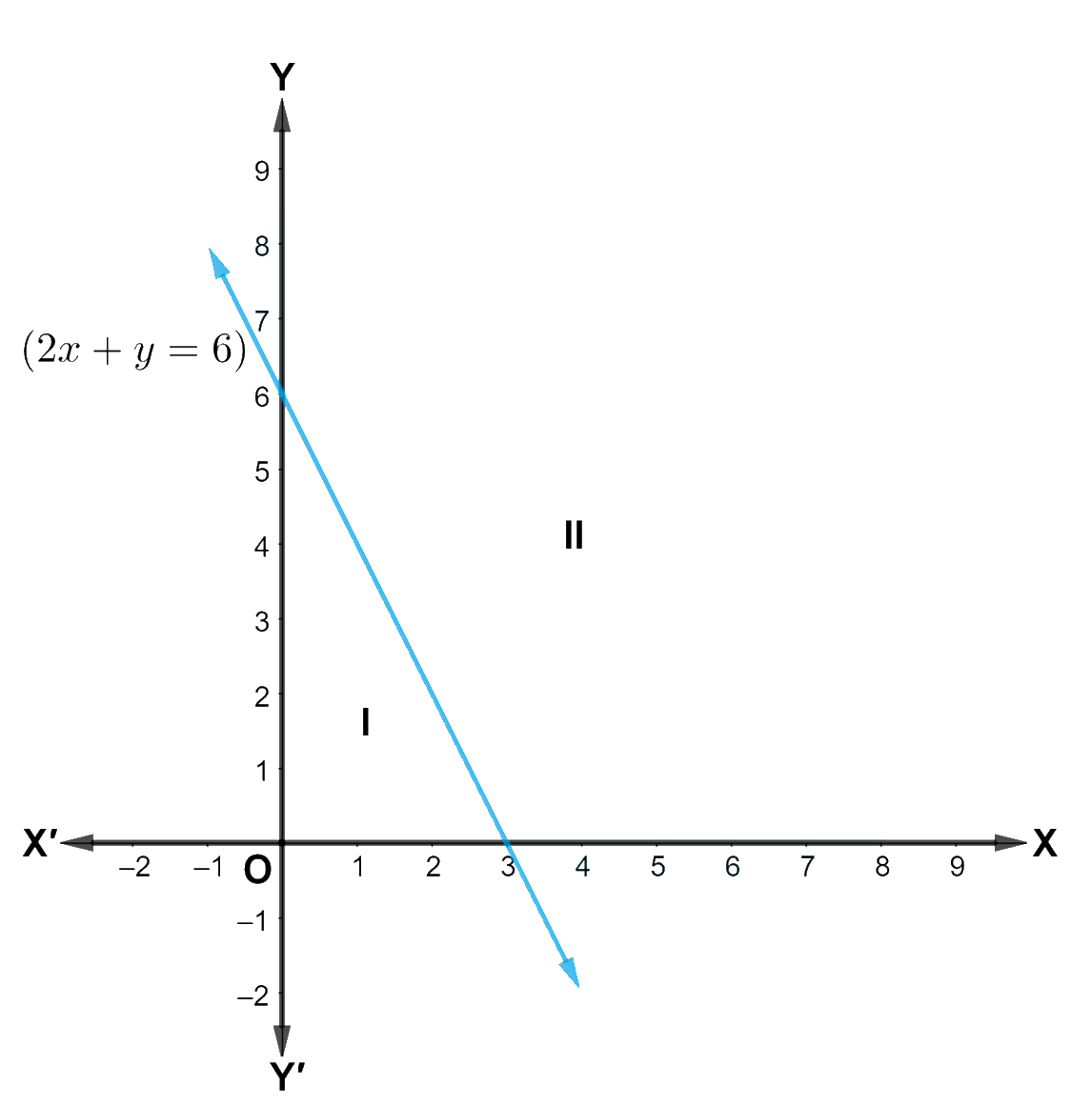## Chapter 6 Ex.6.2 Question 5

Solve the given inequality graphically in two-dimensional plane: $$x - y \le 2$$

### Solution

The graphical representation of $$x - y = 2$$ is given in the figure below.

This line divides the $$xy$$-plane in two half planes.

Select a point (not on the line), which lies in one of the half planes, to determine whether the point satisfies the given inequality or not.

We select the point as $$\left( {0,0} \right)$$.

It is observed that,

$$0 - 0 \le 2$$ or $$0 \le 2,$$ which is true

Therefore, the lower half plane is not the solution region of the given inequality. Also, any point on the line satisfies the given inequality.

Thus, the solution region of the given inequality is the upper half plane containing the points including the line.

The solution region is represented by the shaded region as follows: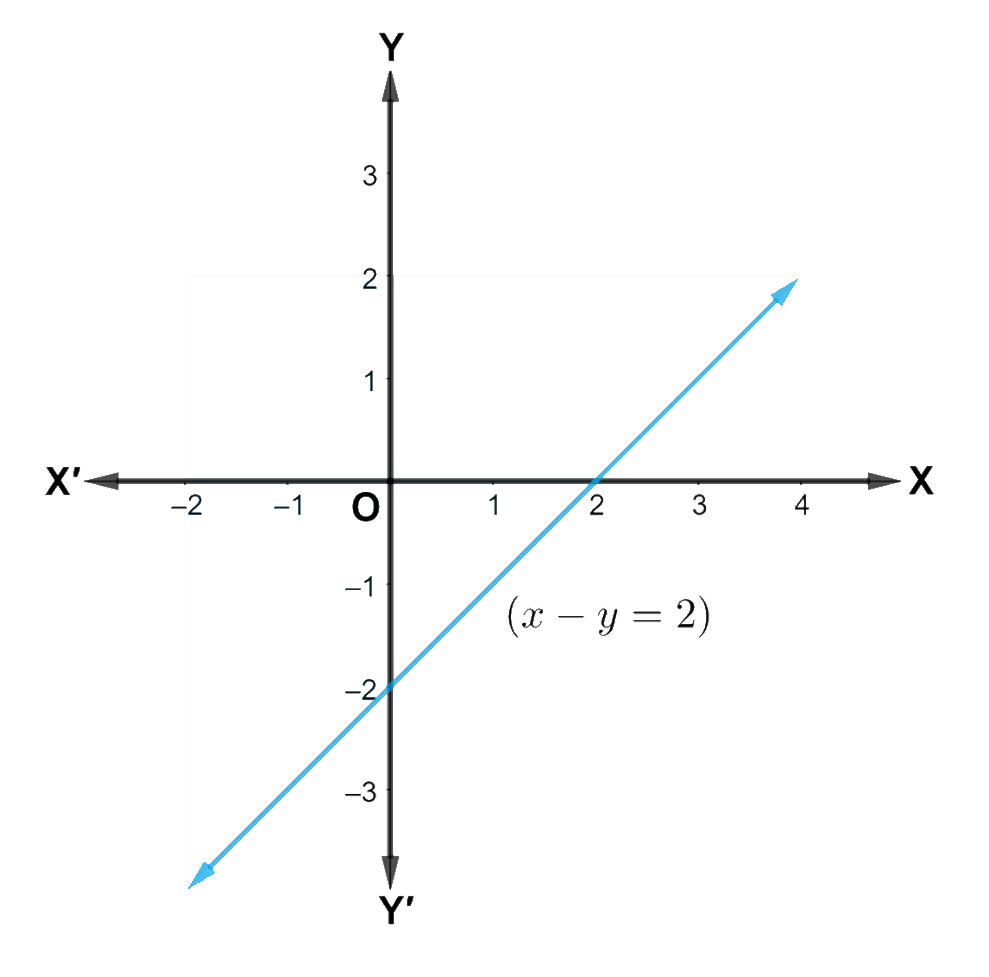## Chapter 6 Ex.6.2 Question 6

Solve the given inequality graphically in two-dimensional plane: $$2x - 3y > 6$$

### Solution

The graphical representation of $$2x - 3y = 6$$ is given as dotted line in the figure below.

This line divides the $$xy-$$plane in two half planes.

Select a point (not on the line), which lies in one of the half planes, to determine whether the point satisfies the given inequality or not.

We select the point as $$\left( {0,0} \right)$$.

It is observed that,

$$2\left( 0 \right) - 3\left( 0 \right) > 6$$ or $$0 > 6$$, which is false

Therefore, the upper half plane is not the solution region of the given inequality. Also, any point on the line does not satisfy the given inequality.

Thus, the solution region of the given inequality is the lower half plane that does not contain the points including the line.

The solution region is represented by the shaded region as follows: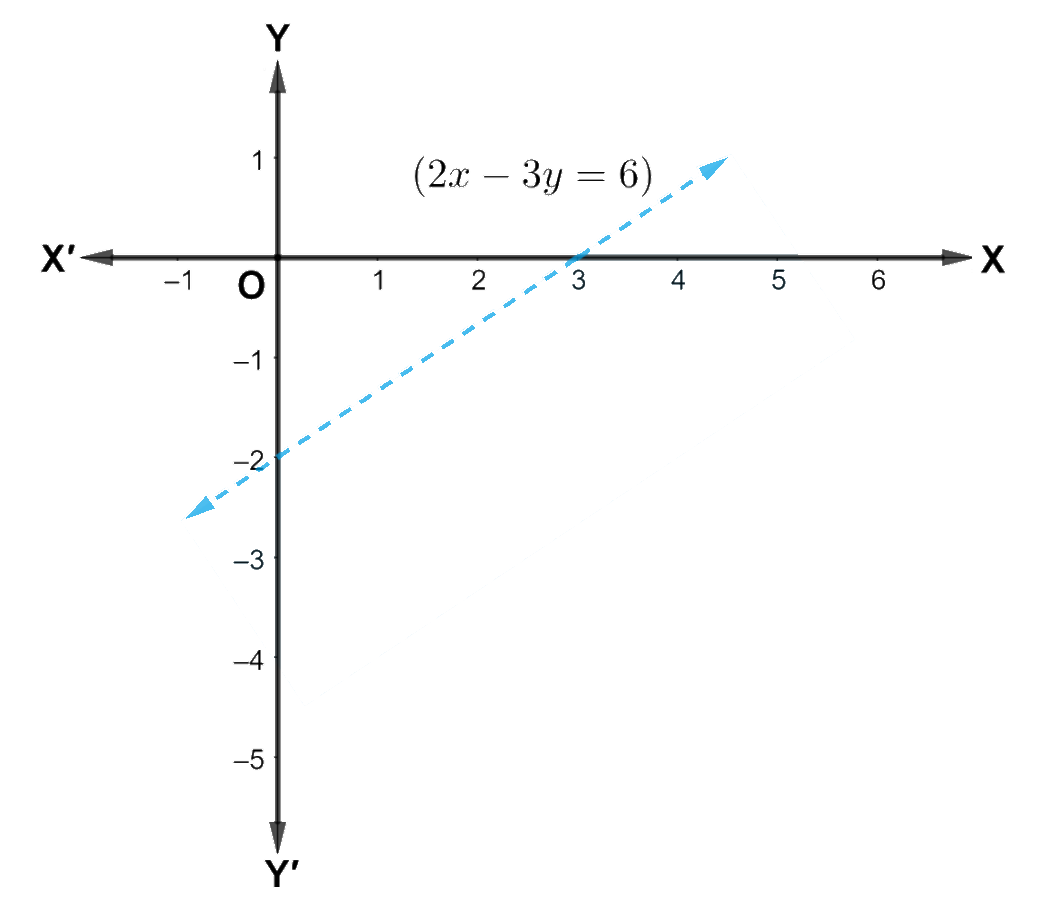## Chapter 6 Ex.6.2 Question 7

Solve the given inequality graphically in two-dimensional plane: $$- 3x + 2y \ge - 6$$

### Solution

The graphical representation of $$- 3x + 2y = - 6$$ is given in the figure below.

This line divides the xy-plane in two half planes.

Select a point (not on the line), which lies in one of the half planes, to determine whether the point satisfies the given inequality or not.

We select the point as $$\left( {0,0} \right)$$.

It is observed that

$$- 3\left( 0 \right) + 2\left( 0 \right) \ge - 6$$ or $$0 \ge - 6,$$ which is true

Therefore, the lower half plane is not the solution region of the given inequality. Also, it is evident that any point on the line satisfies the given inequality.

Thus, the solution region of the given inequality is the half plane containing the points including the line.

The solution region is represented by the shaded region as follows: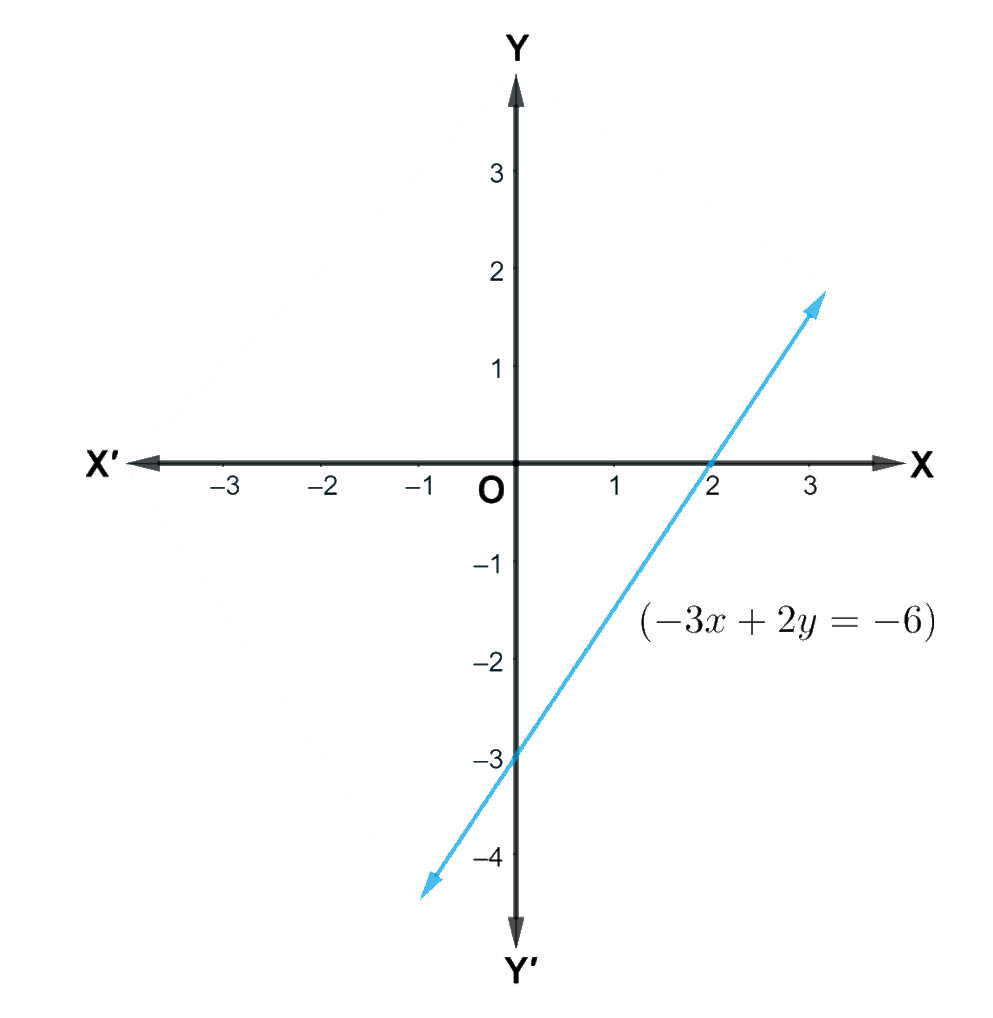## Chapter 6 Ex.6.2 Question 8

Solve the given inequality graphically in two-dimensional plane: $$3y - 5x < 30$$

### Solution

The graphical representation of $$3y - 5x = 30$$ is given as dotted line in the figure below. This line divides the xy-plane in two half planes.

Select a point (not on the line), which lies in one of the half planes, to determine whether the point satisfies the given inequality or not.

We select the point as $$\left( {0,0} \right)$$.

It is observed that,

$$3\left( 0 \right) - 5\left( 0 \right) < 30$$ or $$0 < 30,$$ which is true

Therefore, the upper half plane is not the solution region of the given inequality. Also, it is evident that any point on the line does not satisfy the given inequality.

Thus, the solution region of the given inequality is the half plane containing the point excluding the line.

The solution region is represented by the shaded region as follows: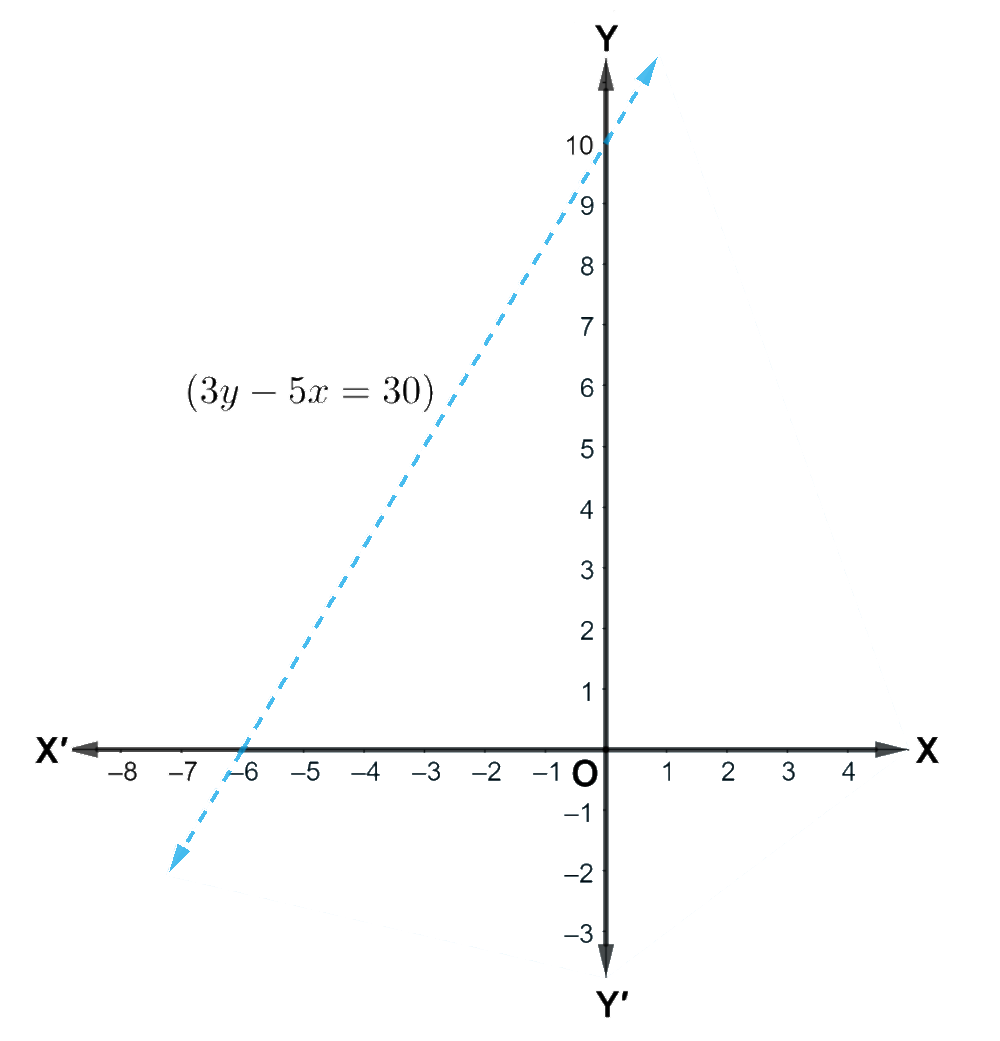## Chapter 6 Ex.6.2 Question 9

Solve the given inequality graphically in two-dimensional plane: $$y < - 2$$

### Solution

The graphical representation of $$y = - 2$$ is given as dotted line in the figure below. This line divides the xy-plane in two half planes.

Select a point (not on the line), which lies in one of the half planes, to determine whether the point satisfies the given inequality or not.

We select the point as $$\left( {0,0} \right)$$.

It is observed that,

$$0 < - 2,$$ which is false

Also, it is evident that any point on the line does not satisfy the given inequality.

Hence, every point below the line, $$y = - 2$$ (excluding all the points on the line), determine the solution of the given inequality.

The solution region is represented by the shaded region as follows: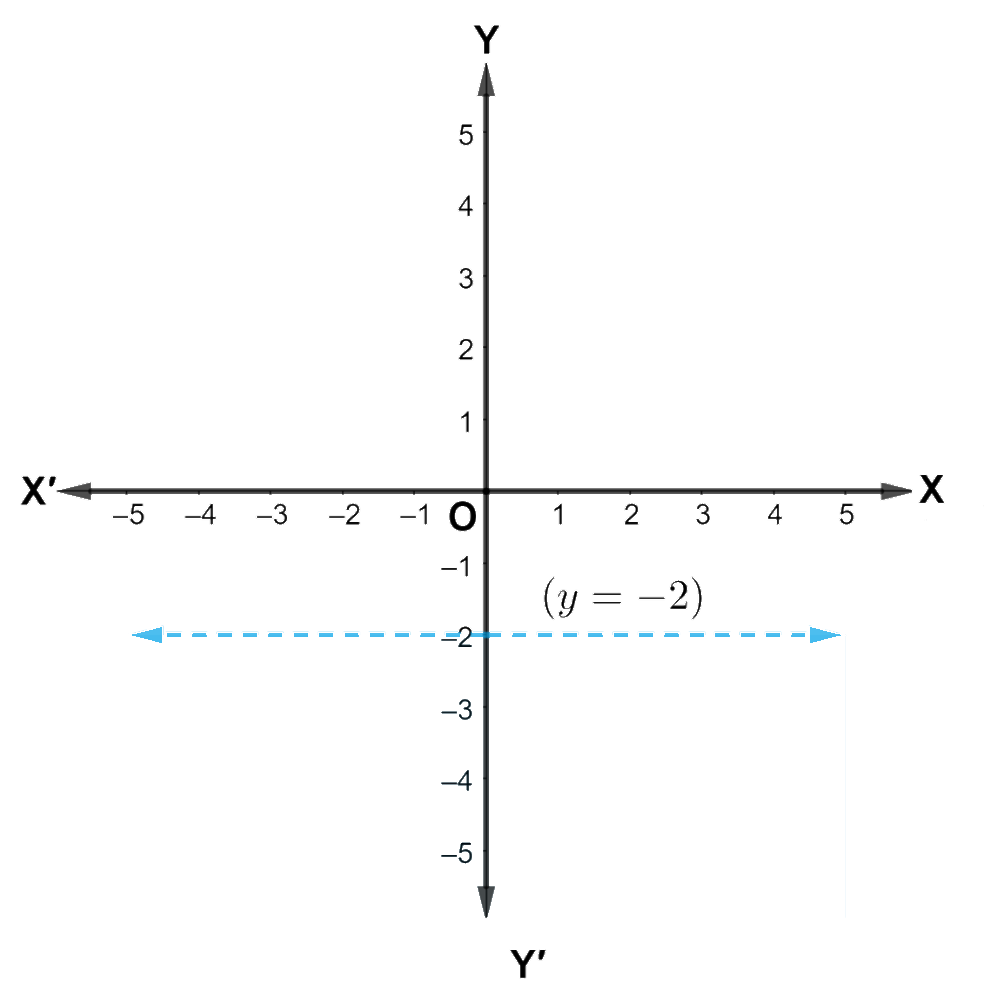## Chapter 6 Ex.6.2 Question 10

Solve the given inequality graphically in two-dimensional plane: $$x > - 3$$

### Solution

The graphical representation of $$x = - 3$$ is given as dotted line in the figure below. This line divides the xy-plane in two half plates.

Select a point (not on the line), which lies in one of the half planes, to determine whether the point satisfies the given inequality or not.

We select the point as $$\left( {0,0} \right)$$.

It is observed that,

$$0 > - 3,$$ which is true

Also, it is evident that any point on the line does not satisfy the given inequality.

Hence, every point on the right side of the line, $$x = - 3$$ (excluding all the points on the line), determines the solution of the given inequality.

The solution region is represented by the shaded region as follows: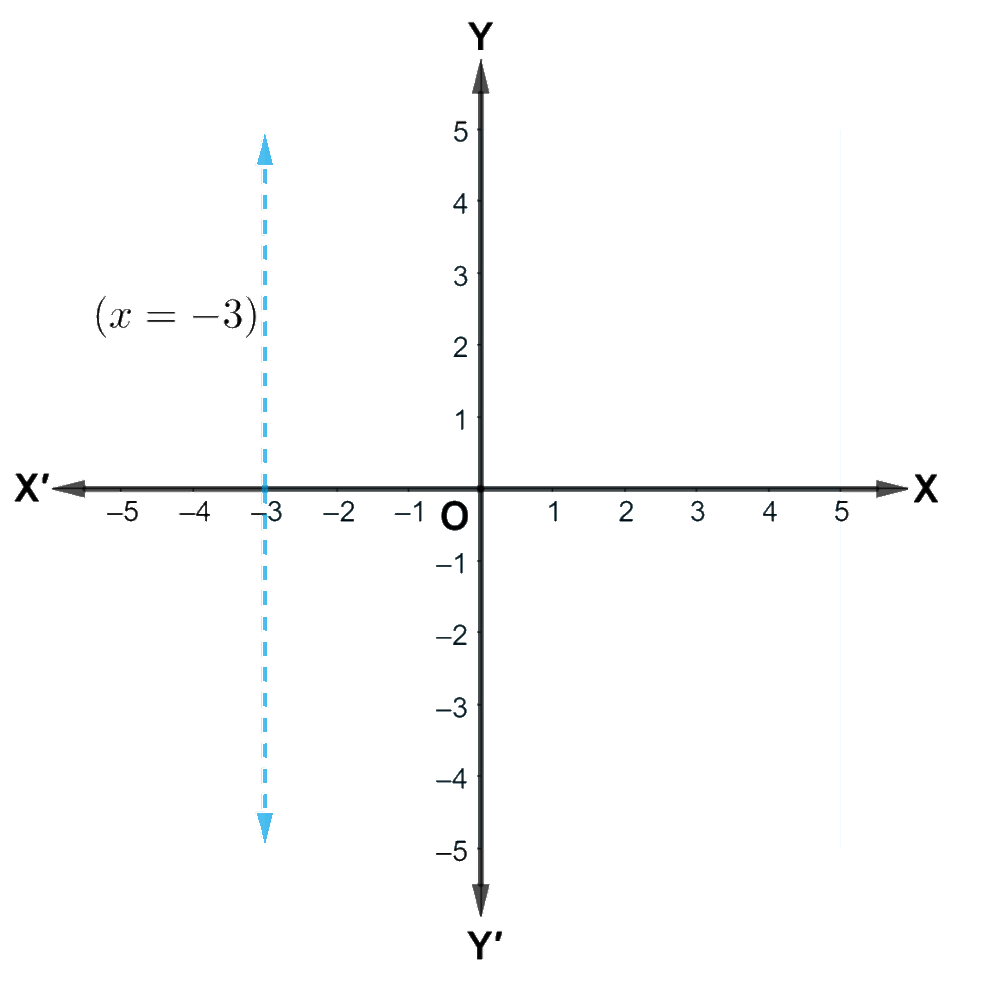Related Sections
Related Sections
Instant doubt clearing with Cuemath Advanced Math Program